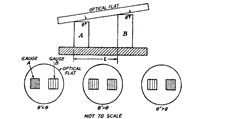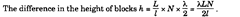# Testing the parallelism of any surface with reference to a standard optically flat surface by using optical flat (Metrology)

6.6.This method is also used for checking height of gauge blocks.
The two blocks, one whose surface is made optically flat and the other whose surface is
to be checked are wrung on a flat reference plane surface. An optical flat is then placed on the
top of both the blocks. In Fig. 6.15, A is the standard reference gauge and B is the gauge blockFig. 6.15. Testing of parallelism.
under test. The distance L between the two gauges is measured very accurately. In case A and
B are perfectly flat, but different in height; then there will be equal wedge shaped air layers
between the gauges A and B, and the optical flat and hence equal number of fringes will be
seen on both the gauge surfaces. In case the surfaces are not parallel, the angles formed by the
surfaces of the two gauges with the optical flat will be different. Let the angles be 8 and 6′
respectively. If the width of the gauge block lis same for both, then number of fringes per unit
width will be different. These possibilities have been shown in Fig. 6.15.
Once it is assured that the surfaces of the two gauges are flat and parallel to each other,
then it is possible to check the height of two gauges also. Let the number of fringes on the
reference gauge block be N in the width of I units.This is however a rough method and meant to explain principle only. For this purpose
the use of interferometer is best.

Next post:

Previous post: